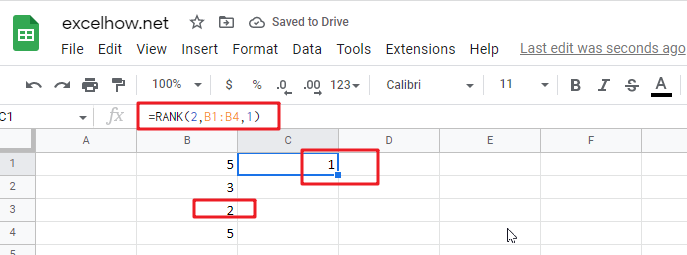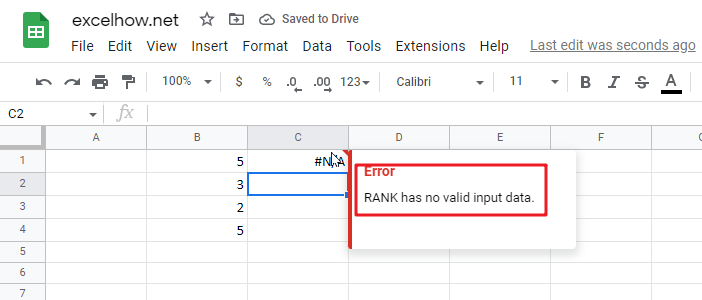# ExcelHow

This article will introduce you how to use the RANK function in google sheets, and will show you a good example to better understand the usage of the RANK function.

## Google Sheets Rank Function Description

The Google Sheets Rank function is used to return the ranking of a given number from a list. If there are duplicate numbers in the list, then the numbers will be in the same rank.

The RANK function is a build-in function in Google Sheets and it is categorized as a Statistical Function.

## Google Sheets Rank Function Syntax

The syntax of the Google Sheets Rank function is explained as follows:

`= RANK (number,reference ,[order])`

Where the arguments of the RANK function are as follows:

• Number – This is a required option, the number that you would like to get ranked
• reference – This is a mandatory option, it may be an array or cell region, or a cell reference contains the specified number
• Order – This is an optional, you can specify the number of 0 or 1 to determine the type of sorting; if the value of order is 1, it means that the RANK function will be sorted in ascending order, otherwise it will be ranked in descending order.

## Google Sheets RANK Function Examples

The below examples will show you how to use Google Sheets RANK Function to get the rank of a given value within a supplied range of cells.

Example 1: If you want to get the sorting of number 2 in a given list B1:B4 in google sheets, then you can use the RANK function to do so with the following formula:

`=RANK(2,B1:B4,1)`Note: The number 2 must be a value that exists in B1:B4, otherwise google sheets will report the error “Rank has no valid input data“.